# Fraction calculator

The calculator performs basic and advanced operations with fractions, expressions with fractions combined with integers, decimals, and mixed numbers. It also shows detailed step-by-step information about the fraction calculation procedure. Solve problems with two, three, or more fractions and numbers in one expression.

## Result:

### 56/7 - 22/3 = 67/21 = 3 4/21 ≅ 3.1904762

Spelled result in words is sixty-seven twenty-firsts (or three and four twenty-firsts).

### How do you solve fractions step by step?

1. Conversion a mixed number 5 6/7 to a improper fraction: 5 6/7 = 5 6/7 = 5 · 7 + 6/7 = 35 + 6/7 = 41/7

To find new numerator:
a) Multiply the whole number 5 by the denominator 7. Whole number 5 equally 5 * 7/7 = 35/7
b) Add the answer from previous step 35 to the numerator 6. New numerator is 35 + 6 = 41
c) Write a previous answer (new numerator 41) over the denominator 7.

Five and six sevenths is forty-one sevenths
2. Conversion a mixed number 2 2/3 to a improper fraction: 2 2/3 = 2 2/3 = 2 · 3 + 2/3 = 6 + 2/3 = 8/3

To find new numerator:
a) Multiply the whole number 2 by the denominator 3. Whole number 2 equally 2 * 3/3 = 6/3
b) Add the answer from previous step 6 to the numerator 2. New numerator is 6 + 2 = 8
c) Write a previous answer (new numerator 8) over the denominator 3.

Two and two thirds is eight thirds
3. Subtract: 41/7 - 8/3 = 41 · 3/7 · 3 - 8 · 7/3 · 7 = 123/21 - 56/21 = 123 - 56/21 = 67/21
For adding, subtracting, and comparing fractions, it is suitable to adjust both fractions to a common (equal, identical) denominator. The common denominator you can calculate as the least common multiple of both denominators - LCM(7, 3) = 21. In practice, it is enough to find the common denominator (not necessarily the lowest) by multiplying the denominators: 7 × 3 = 21. In the next intermediate step, the fraction result cannot be further simplified by canceling.
In words - forty-one sevenths minus eight thirds = sixty-seven twenty-firsts.

#### Rules for expressions with fractions:

Fractions - use the slash “/” between the numerator and denominator, i.e., for five-hundredths, enter 5/100. If you are using mixed numbers, be sure to leave a single space between the whole and fraction part.
The slash separates the numerator (number above a fraction line) and denominator (number below).

Mixed numerals (mixed fractions or mixed numbers) write as non-zero integer separated by one space and fraction i.e., 1 2/3 (having the same sign). An example of a negative mixed fraction: -5 1/2.
Because slash is both signs for fraction line and division, we recommended use colon (:) as the operator of division fractions i.e., 1/2 : 3.

Decimals (decimal numbers) enter with a decimal point . and they are automatically converted to fractions - i.e. 1.45.

The colon : and slash / is the symbol of division. Can be used to divide mixed numbers 1 2/3 : 4 3/8 or can be used for write complex fractions i.e. 1/2 : 1/3.
An asterisk * or × is the symbol for multiplication.
Plus + is addition, minus sign - is subtraction and ()[] is mathematical parentheses.
The exponentiation/power symbol is ^ - for example: (7/8-4/5)^2 = (7/8-4/5)2

#### Examples:

subtracting fractions: 2/3 - 1/2
multiplying fractions: 7/8 * 3/9
dividing Fractions: 1/2 : 3/4
exponentiation of fraction: 3/5^3
fractional exponents: 16 ^ 1/2
adding fractions and mixed numbers: 8/5 + 6 2/7
dividing integer and fraction: 5 ÷ 1/2
complex fractions: 5/8 : 2 2/3
decimal to fraction: 0.625
Fraction to Decimal: 1/4
Fraction to Percent: 1/8 %
comparing fractions: 1/4 2/3
multiplying a fraction by a whole number: 6 * 3/4
square root of a fraction: sqrt(1/16)
reducing or simplifying the fraction (simplification) - dividing the numerator and denominator of a fraction by the same non-zero number - equivalent fraction: 4/22
expression with brackets: 1/3 * (1/2 - 3 3/8)
compound fraction: 3/4 of 5/7
fractions multiple: 2/3 of 3/5
divide to find the quotient: 3/5 ÷ 2/3

The calculator follows well-known rules for order of operations. The most common mnemonics for remembering this order of operations are:
PEMDAS - Parentheses, Exponents, Multiplication, Division, Addition, Subtraction.
BEDMAS - Brackets, Exponents, Division, Multiplication, Addition, Subtraction
BODMAS - Brackets, Of or Order, Division, Multiplication, Addition, Subtraction.
GEMDAS - Grouping Symbols - brackets (){}, Exponents, Multiplication, Division, Addition, Subtraction.
Be careful, always do multiplication and division before addition and subtraction. Some operators (+ and -) and (* and /) has the same priority and then must evaluate from left to right.

## Fractions in word problems:

• CupcakesIn a bowl was some cupcakes. Janka ate one third and Danka ate one quarter of cupcakes. a) How many of cookies ate together? b) How many cookies remain in a bowl? Write the results as a decimal number and in notepad also as a fraction.
• Algebra problemThis is algebra. Let n represent an unknown number. 1. Eight more than the number n 2. Three times the number n 3. The product of the number n and eight 4. Three less than the number n 5. Three decreased by the number n
• Toilets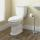Federal law requires that all residential toilets sold in the United States use no more than 1 3/5 gallons of water per flush. Before this legislation, conventional toilets used 3 2/5 gallons of water per flush. Find the amount of water saved in one year
• SavingsEva borrowed 1/3 of her savings to her brother, 1/2 of savings spent in the store and 7 euros left. How much did she save?
• Square metal sheetWe cut out four squares of 300 mm side from a square sheet metal plate with a side of 0,7 m. Express the fraction and the percentage of waste from the square metal sheet.
• Difference of two fractions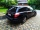What is the difference between 1/2 and 1/6? (Write the answer as a fraction in lowest terms. )
• Package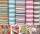The package was 23 meters of textile. The first day sold 12.3 meters. How many meters of textile remained in the package?
• Evaluate - lowest termsEvaluate: 16/25 - 11/25 (Express answer as a fraction reduced to lowest terms. )
• MichaelMichael had a bar of chocolate. He ate 1/2 of it and gave away 1/3. What fraction had he left?
• You leaveYou leave school and the end of the day and walk 3/8 of a mile away before realizing that you left your backpack and immediately turn around you then walk 1/6 of a mile back towards school at this point assuming you walked in a straight line how many mile
• Akpan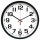Akpan spent 3/8 of his time in school during the week. What fraction of his time does he spend at home during the week?
• In bucket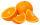In bucket of 87 oranges 1/3 are spoiled ones and the remaining are eaten by the member of a family. How many oranges are eaten by the member of family?
• Human 3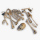Human bones are 3/10 living tissues 9/20 minerals, and the rest water. What fraction of human bone is water?